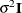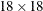### Clustered Data Example

Consider the following SAS data set as an introductory example:

data heights;
input Family Gender\$ Height @@;
datalines;
1 F 67   1 F 66   1 F 64   1 M 71   1 M 72   2 F 63
2 F 63   2 F 67   2 M 69   2 M 68   2 M 70   3 F 63
3 M 64   4 F 67   4 F 66   4 M 67   4 M 67   4 M 69
;


The response variable Height measures the heights (in inches) of 18 individuals. The individuals are classified according to Family and Gender. You can perform a traditional two-way analysis of variance of these data with the following PROC MIXED statements:

proc mixed data=heights;
class Family Gender;
model Height = Gender Family Family*Gender;
run;


The PROC MIXED statement invokes the procedure. The CLASS statement instructs PROC MIXED to consider both Family and Gender as classification variables. Dummy (indicator) variables are, as a result, created corresponding to all of the distinct levels of Family and Gender. For these data, Family has four levels and Gender has two levels.

The MODEL statement first specifies the response (dependent) variable Height. The explanatory (independent) variables are then listed after the equal (=) sign. Here, the two explanatory variables are Gender and Family, and these are the main effects of the design. The third explanatory term, Family*Gender, models an interaction between the two main effects.

PROC MIXED uses the dummy variables associated with Gender, Family, and Family*Gender to construct thematrix for the linear model. A column of 1s is also included as the first column ofto model a global intercept. There are noormatrices for this model, andis assumed to equal, whereis anidentity matrix.

The RUN statement completes the specification. The coding is precisely the same as with the GLM procedure. However, much of the output from PROC MIXED is different from that produced by PROC GLM.

The output from PROC MIXED is shown in Figure 59.1Figure 59.7.

The Model Information table in Figure 59.1 describes the model, some of the variables that it involves, and the method used in fitting it. This table also lists the method (profile, factor, parameter, or none) for handling the residual variance.

Figure 59.1: Model Information

The Mixed Procedure

Model Information
Data Set WORK.HEIGHTS
Dependent Variable Height
Covariance Structure Diagonal
Estimation Method REML
Residual Variance Method Profile
Fixed Effects SE Method Model-Based
Degrees of Freedom Method Residual

The Class Level Information table in Figure 59.2 lists the levels of all variables specified in the CLASS statement. You can check this table to make sure that the data are correct.

Figure 59.2: Class Level Information

Class Level Information
Class Levels Values
Family 4 1 2 3 4
Gender 2 F M

The Dimensions table in Figure 59.3 lists the sizes of relevant matrices. This table can be useful in determining CPU time and memory requirements.

Figure 59.3: Dimensions

Dimensions
Covariance Parameters 1
Columns in X 15
Columns in Z 0
Subjects 1
Max Obs Per Subject 18

The Number of Observations table in Figure 59.4 displays information about the sample size being processed.

Figure 59.4: Number of Observations

Number of Observations
Number of Observations Used 18
Number of Observations Not Used 0

The Covariance Parameter Estimates table in Figure 59.5 displays the estimate offor the model.

Figure 59.5: Covariance Parameter Estimates

Covariance Parameter Estimates
Cov Parm Estimate
Residual 2.1000

The Fit Statistics table in Figure 59.6 lists several pieces of information about the fitted mixed model, including values derived from the computed value of the restricted/residual likelihood.

Figure 59.6: Fit Statistics

Fit Statistics
-2 Res Log Likelihood 41.6
AIC (smaller is better) 43.6
AICC (smaller is better) 44.1
BIC (smaller is better) 43.9

The Type 3 Tests of Fixed Effects table in Figure 59.7 displays significance tests for the three effects listed in the MODEL statement. The Type 3 F statistics and p-values are the same as those produced by the GLM procedure. However, because PROC MIXED uses a likelihood-based estimation scheme, it does not directly compute or display sums of squares for this analysis.

Figure 59.7: Tests of Fixed Effects

Type 3 Tests of Fixed Effects
Effect Num DF Den DF F Value Pr > F
Gender 1 10 17.63 0.0018
Family 3 10 5.90 0.0139
Family*Gender 3 10 2.89 0.0889

The Type 3 test for Family*Gender effect is not significant at the 5% level, but the tests for both main effects are significant.

The important assumptions behind this analysis are that the data are normally distributed and that they are independent with constant variance. For these data, the normality assumption is probably realistic since the data are observed heights. However, since the data occur in clusters (families), it is very likely that observations from the same family are statistically correlated—that is, not independent.

The methods implemented in PROC MIXED are still based on the assumption of normally distributed data, but you can drop the assumption of independence by modeling statistical correlation in a variety of ways. You can also model variances that are heterogeneous—that is, nonconstant.

For the height data, one of the simplest ways of modeling correlation is through the use of random effects. Here the family effect is assumed to be normally distributed with zero mean and some unknown variance. This is in contrast to the previous model in which the family effects are just constants, or fixed effects. Declaring Family as a random effect sets up a common correlation among all observations having the same level of Family.

Declaring Family*Gender as a random effect models an additional correlation between all observations that have the same level of both Family and Gender. One interpretation of this effect is that a female in a certain family exhibits more correlation with the other females in that family than with the other males, and likewise for a male. With the height data, this model seems reasonable.

The statements to fit this correlation model in PROC MIXED are as follows:

proc mixed;
class Family Gender;
model Height = Gender;
random Family Family*Gender;
run;


Note that Family and Family*Gender are now listed in the RANDOM statement. The dummy variables associated with them are used to construct thematrix in the mixed model. Thematrix now consists of a column of 1s and the dummy variables for Gender.

Thematrix for this model is diagonal, and it contains the variance components for both Family and Family*Gender. Thematrix is still assumed to equal, whereis an identity matrix.

The output from this analysis is as follows.

Figure 59.8: Model Information

The Mixed Procedure

Model Information
Data Set WORK.HEIGHTS
Dependent Variable Height
Covariance Structure Variance Components
Estimation Method REML
Residual Variance Method Profile
Fixed Effects SE Method Model-Based
Degrees of Freedom Method Containment

The Model Information table in Figure 59.8 shows that the containment method is used to compute the degrees of freedom for this analysis. This is the default method when a RANDOM statement is used; see the description of the DDFM= option for more information.

Figure 59.9: Class Level Information

Class Level Information
Class Levels Values
Family 4 1 2 3 4
Gender 2 F M

The Class Level Information table in Figure 59.9 is the same as before. The Dimensions table in Figure 59.10 displays the new sizes of theandmatrices.

Figure 59.10: Dimensions and Number of Observations

Dimensions
Covariance Parameters 3
Columns in X 3
Columns in Z 12
Subjects 1
Max Obs Per Subject 18

Number of Observations
Number of Observations Used 18
Number of Observations Not Used 0

The Iteration History table in Figure 59.11 displays the results of the numerical optimization of the restricted/residual likelihood. Six iterations are required to achieve the default convergence criterion of 1E–8.

Figure 59.11: REML Estimation Iteration History

Iteration History
Iteration Evaluations -2 Res Log Like Criterion
0 1 74.11074833
1 2 71.51614003 0.01441208
2 1 71.13845990 0.00412226
3 1 71.03613556 0.00058188
4 1 71.02281757 0.00001689
5 1 71.02245904 0.00000002
6 1 71.02245869 0.00000000

 Convergence criteria met.

The Covariance Parameter Estimates table in Figure 59.12 displays the results of the REML fit. The Estimate column contains the estimates of the variance components for Family and Family*Gender, as well as the estimate of.

Figure 59.12: Covariance Parameter Estimates (REML)

Covariance Parameter Estimates
Cov Parm Estimate
Family 2.4010
Family*Gender 1.7657
Residual 2.1668

The Fit Statistics table in Figure 59.13 contains basic information about the REML fit.

Figure 59.13: Fit Statistics

Fit Statistics
-2 Res Log Likelihood 71.0
AIC (smaller is better) 77.0
AICC (smaller is better) 79.0
BIC (smaller is better) 75.2

The Type 3 Tests of Fixed Effects table in Figure 59.14 contains a significance test for the lone fixed effect, Gender. Note that the associated p-value is not nearly as significant as in the previous analysis. This illustrates the importance of correctly modeling correlation in your data.

Figure 59.14: Type 3 Tests of Fixed Effects

Type 3 Tests of Fixed Effects
Effect Num DF Den DF F Value Pr > F
Gender 1 3 7.95 0.0667

An additional benefit of the random effects analysis is that it enables you to make inferences about gender that apply to an entire population of families, whereas the inferences about gender from the analysis where Family and Family*Gender are fixed effects apply only to the particular families in the data set.

PROC MIXED thus offers you the ability to model correlation directly and to make inferences about fixed effects that apply to entire populations of random effects.